# AP Physics 1 : Wavelength and Amplitude

## Example Questions

### Example Question #1 : Wavelength And Amplitude

A severe storm has moved into your area and the weather sirens have begun to go off. The frequency of the siren ranges between 200 hz and 2,000 hz. What is the range of wavelength of the emitted sound waves?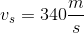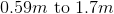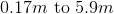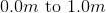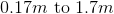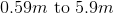Explanation:

We can use the following equation to solve this problem: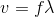where

v = velocity of sound

f = frequency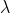= wavelength

Rearranging for wavelength, we get: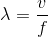Plugging in our values, we get: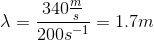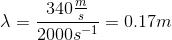You do not need to have this equation memorized in order to solve this problem. A very useful skill in physics is being able to solve problems based solely off your units.

We need to have an answer with the units of meters. We are given units of m/s and 1/s. How can we go from these to meters? Simply divide the value with the units of m/s by the value with the units of 1/s. This gives you an answer with the units of meters. Once written out, this is the final equation written above. No need to spend extra time memorizing the equation!

### Example Question #2 : Wavelength And Amplitude

What is the wavelength of a radio station that broadcasts at 92.9MHz?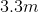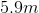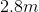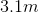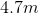Explanation:

We need to know the speed of light, which is: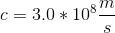We can use this to calculate the wavelength of any electromagnetic wave if we know the frequency, using the equation:If you're unsure of how to come about this equation, you should be able to derive it by looking at your units. Use our given values to solve for the wavelength: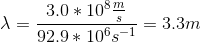### Example Question #3 : Wavelength And Amplitude

A wave with a speed of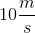has a frequency of 30Hz. What is the wavelength?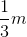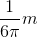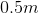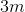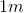Explanation:

Since the wave has a frequency of 30Hz, we know it completes 30 cycles every second. As its velocity is, we also know that in that second, the wave has moved 10m. Dividing the total distance, 10m, by the number of cycles, 30, we get the number of meters travelled in each cycle which is the wavelength: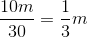### Example Question #4 : Wavelength And Amplitude

Given the speed of a wave as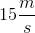and the wavelength of 0.2m, find its frequency.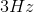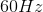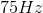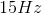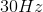Explanation:

Speed is frequency times wavelength. So the frequency must be speed divided by wavelength. In this case: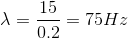This makes sense as in one second, the wave will have travelled 15m. Each meter is 5 cycles, so over the distance the wave has travelled in one second, it has completed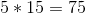cycles.

### Example Question #5 : Wavelength And Amplitude

An equation of a wave is given by the following formula: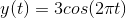Here,is given in meters andis given in seconds. Determine the period of the wave in seconds.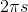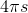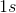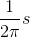Explanation:

A trigonometric equation given by the following formulaHere,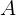is given in meters,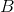is given in Hertz,is given in seconds, and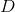is given in meters. The period is given by: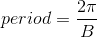In our case: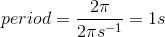### Example Question #6 : Wavelength And Amplitude

The music we listen to on the radio is transmitted in the form of radio waves. You might have seen that a radio station on FM is broadcasted in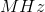, which is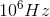What is the wavelength of a radio wave if it's being transmitted on a frequency of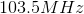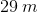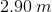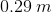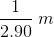Explanation:

Radio waves travel at the speed of light. The relationship between wavelength, frequency, and wave speed is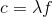, whereis the wavelength andis the frequency.

In our case,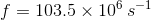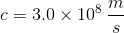Therefore,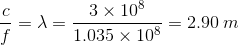### Example Question #7 : Wavelength And Amplitude

An original signal's frequency is halved by a resistor, and no other part of the wave is effected. Determine the change in the amplitude of the signal.

The amplitude is doubled

The amplitude is unchanged

The amplitude is halved